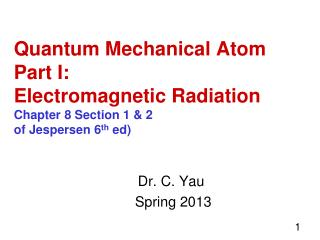DownloadDownload PresentationDr. C. Yau Spring 2013

# Dr. C. Yau Spring 2013

Télécharger la présentation## Dr. C. Yau Spring 2013

- - - - - - - - - - - - - - - - - - - - - - - - - - - E N D - - - - - - - - - - - - - - - - - - - - - - - - - - -
##### Presentation Transcript

1. Quantum Mechanical AtomPart I:Electromagnetic RadiationChapter 8 Section 1 & 2of Jespersen 6th ed) Dr. C. Yau Spring 2013

2. Electromagnetic Radiation • Electromagnetic radiation carries E thru space by means of a wave. • Properties of a wave: (Refer to lec. Notes) • Wavelength (, pronounced lambda) • Frequency (, pronounced nu) • Amplitude (A) • Energy of the photon (uniform packets of E, proportional to frequency)

3. Units of Measurement Wavelength: m, cm, m, nm, angstrom = Å 1 nm = 10 Å 1 m = 1010 Å Frequency: cycles per second = 1/sec = sec-1 1 Hertz = = 1 Hz =1 sec-1 Energy: J, kJ, ergs

4. How are they related?  x  = c = 2.998x108 m s-1 (speed of light)  is inversely proportional to  Example 8.1 p. 253 Mycobacterium tuberculosis, the organism that causes tuberculosis, can be completely destroyed by irradiation with UV light with a wavelength of 254 nm. What is the frequency of this radiation?

5. Example 8.2 p. 308 Radio station WKXR is an AM radio station broadcasting from Asheboro, N Carolina, at a frequency of 1260 kHz. What is the wavelength of these radio waves expressed in meters? Do Pract Exer 1, 2, 3 p.308

6. Energy of a Light Wave The energy carried by a light wave is said to be in “discrete” packets called "photons." Size of energy of the photons is proportional to the frequency of the wave. E = h  where h = Planck's constant = 6.626x10-34 J s You don’t need to memorize constants.

7. The “Rainbow” • The rainbow is a small region of the electromagnetic radiation spectrum, the “visible” region of the spectrum. • You should know the names of the rest of the regions of the spectrum, and in order of energy, frequency and wavelength. (See lecture notes.)

8. Electromagnetic Radiation Spectrum Fig. 8.3 p. 310

9. Absorption of light by chlorophyll

10. What is the energy of a photon of the red light at 700. nm? • What do we know about energy and the wave? • How is wavelength related to frequency? • How is energy related to wavelength?

11. Continuous vs. Line Spectrum What do we see if ordinary white light is passed through a prism? If we pass a current through a partially evacuated tube of a gas, a light is emitted. If this emitted light is passed through a prism, we do not see a continuous rainbow, instead we see...

12. Figure 8.7 p. 314 Emission Line Spectrum We see discrete colored lines on a black background

13. Line Spectra are like fingerprints

14. Line Spectrum of H2 A mathematician came up with an equation that describes the pattern of lines observed for hydrogen (the Rydberg Eqn). The equation does not EXPLAIN why there is such a pattern. Bohr came up with his Planetary Model of the Atom that EXPLAINS why hydrogen would produce such a pattern of lines. (See lecture notes.)

15. Bohr's Calculations Each emission line of hydrogen is due to the energy released in the transition of an electron from a higher E level to a lower one. Bohr's equation En =  B/n2 Bohr's constant, B = 2.180x10-18 J This allows us to calculate the E of each level. The amount of E released is equal to ΔE. ΔE = Ehi – Elo Once we have calculated ΔE, we can determine the corresponding  and . (See lecture notes.)

16. Why Schroedinger has to come up with a different model: Bohr’s theory could predict emission lines for one electron system ONLY. What are one-electron systems? How is Schroedinger’s model different from Bohr’s? See next PowerPoint.